Function Repository Resource:

# FindListProof

Try to find a proof of equivalence between lists in a given multiway system

Contributed by: Jonathan Gorard
 ResourceFunction["FindListProof"][thm,axms] tries to find a proof of the list equivalence theorem thm using the multiway system axioms axms.

## Details and Options

If ResourceFunction["FindListProof"][thm,axms] succeeds in deriving the theorem thm from the axioms axms, then it returns a ProofObject expression. If it succeeds in showing that the theorem cannot be derived from the axioms, it returns a Failure object. Otherwise, it may not terminate unless time constrained.
ResourceFunction["FindListProof"] accepts both individual axioms and lists of axioms, and likewise for theorems.
ResourceFunction["FindListProof"] has the following option:
 TimeConstraint Infinity how much time to allow
If ResourceFunction["FindListProof"] exceeds the specified time constraint, it returns a Failure object.
ResourceFunction["FindListProof"][thm,axms] uses the KnuthBendix completion algorithm to prove that the theorem thm follows from the axioms axms.

## Examples

### Basic Examples (2)

Prove an elementary theorem regarding the equivalence of two lists in a simple multiway system:

 In:=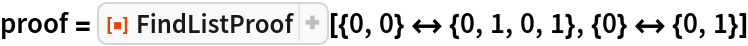Out=Show the abstract proof network, with tooltips showing the intermediate expressions:

 In:=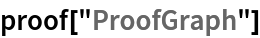Out=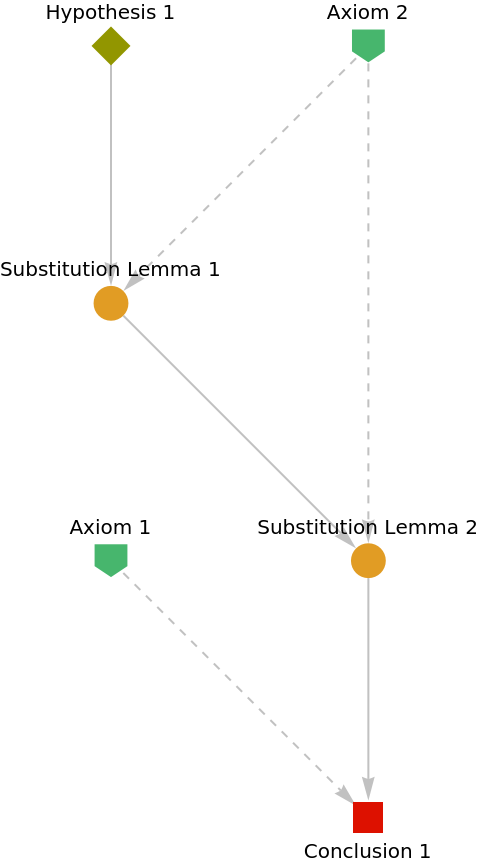Show the complete list of proof steps as a Dataset object:

 In:=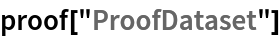Out=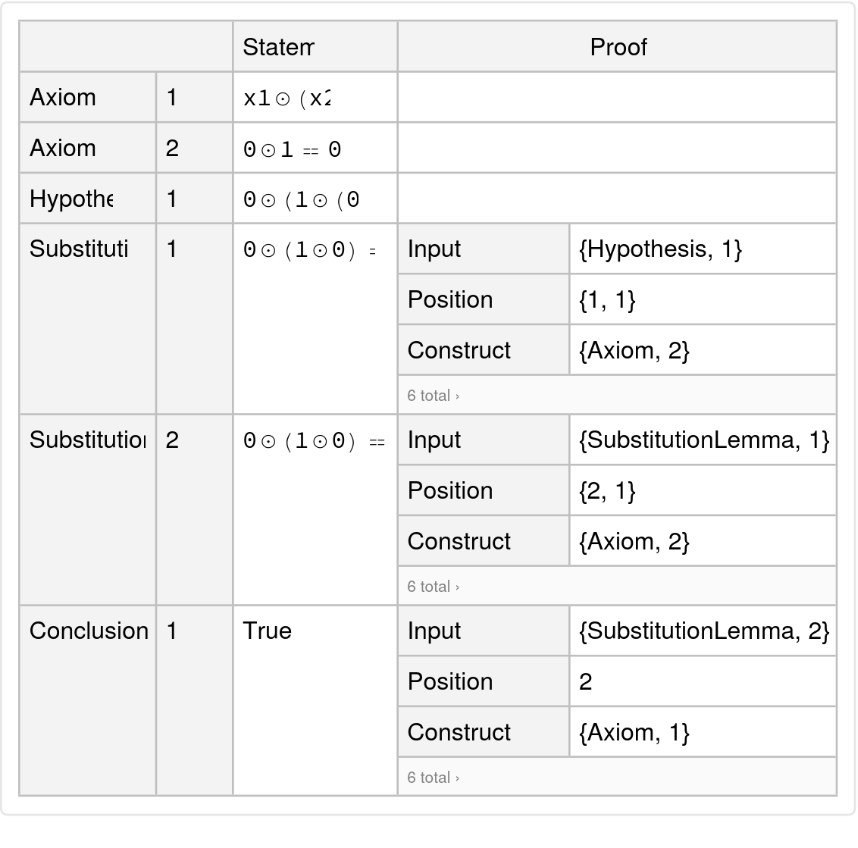Typeset a natural language argument:

 In:=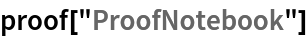Out=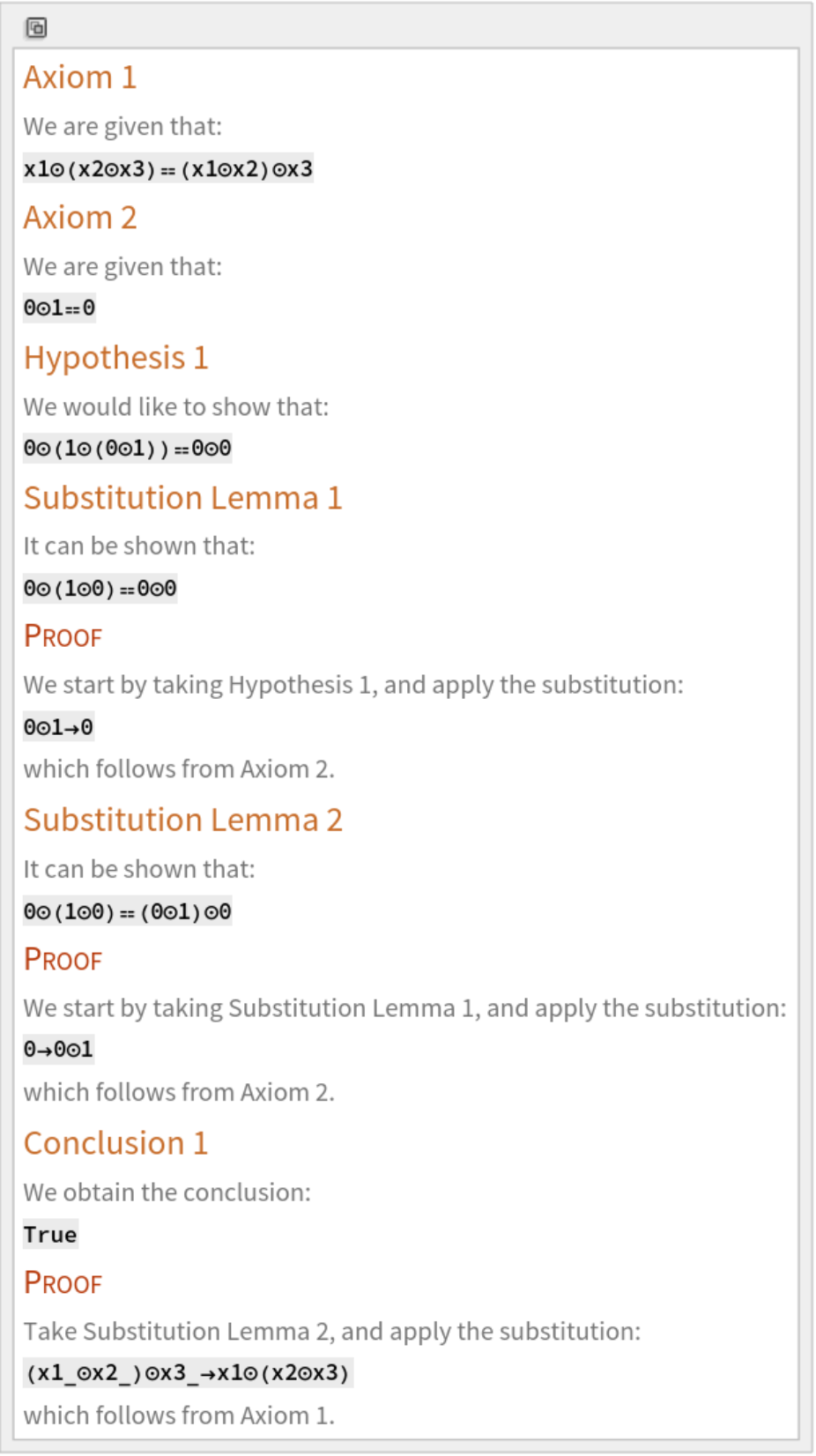Prove a more sophisticated theorem involving multiple rules and hypotheses:

 In:=Out=Show the abstract proof network:

 In:=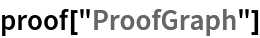Out=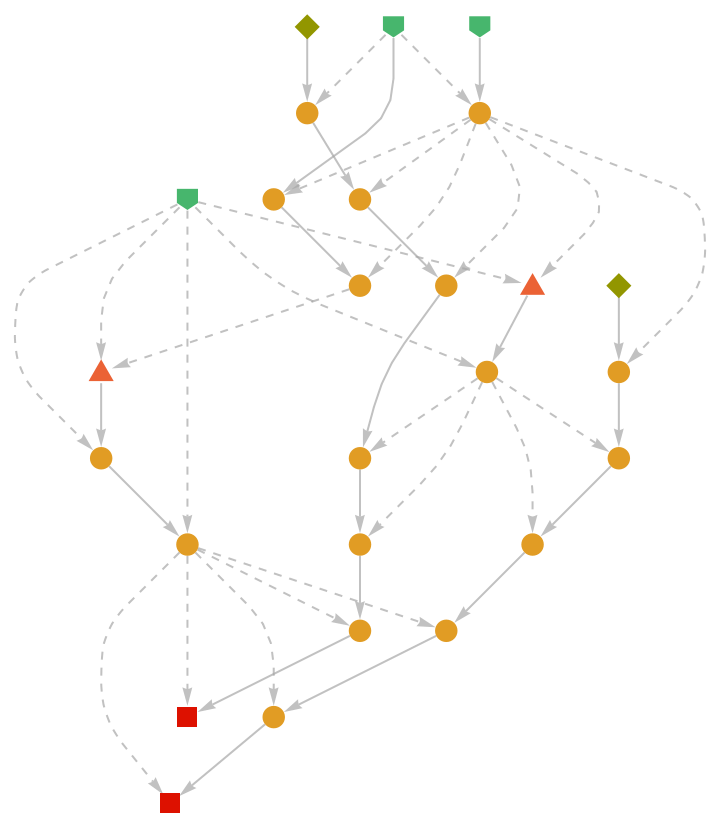### Scope (2)

Show that a list equivalence proposition cannot be derived from a given set of multiway system axioms:

 In:=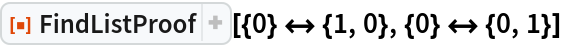Out=#### Rules and Initial Conditions (2)

FindListProof accepts both individual axioms and lists of axioms:

 In:=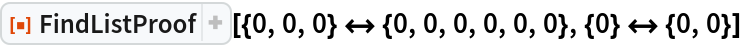Out=In:=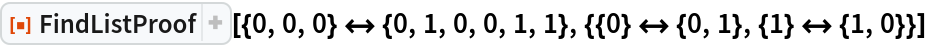Out=Likewise for theorems:

 In:=Out=### Options (2)

#### TimeConstraint (2)

Use to limit the computation time to t seconds:

 In:=Out=By default, FindListProof looks for a proof indefinitely:

 In:=Out=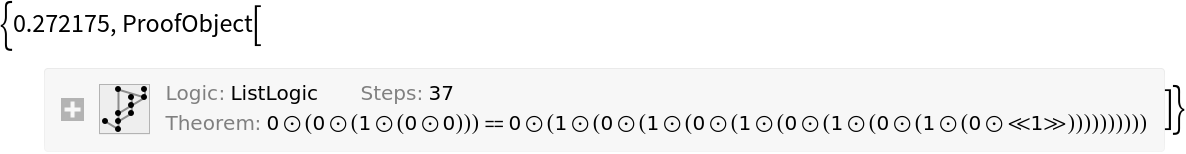### Properties and Relations (1)

FindListProof will return a proof object for a particular theorem if and only if the associated path exists in the corresponding multiway system:

 In:=Out=In:=Out=In:=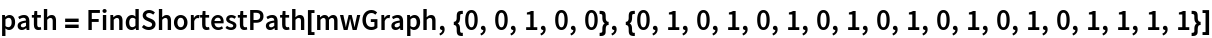Out=In:=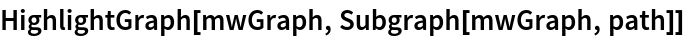Out=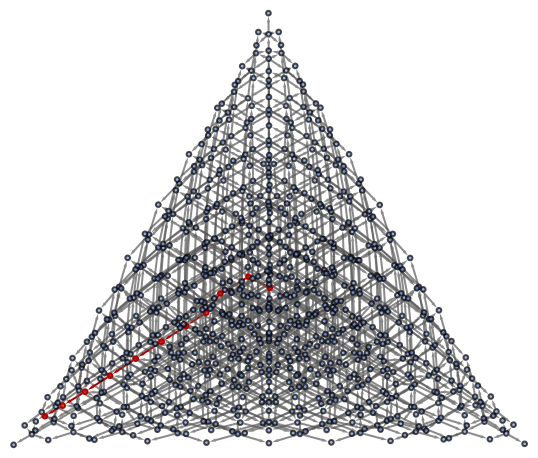Jonathan Gorard

## Version History

• 1.0.0 – 03 August 2020